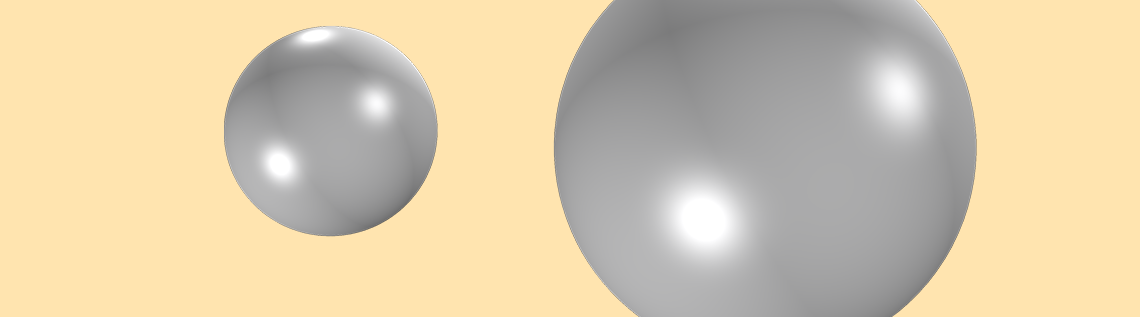# How to Calculate a Capacitance Matrix in COMSOL Multiphysics®

June 7, 2017

Capacitance calculations in the COMSOL Multiphysics® software seem easy. If you only have two conductors, the recipe is simple: Take one conductor and set it to grounded, set the other as a terminal, and compute the solution. Then, a built-in variable delivers the capacitance. But what if you have more than two conductors, like in touchscreens, transmission lines, and capacitive sensors? If standard textbook terminology has you lost, follow along with this working example of calculating a capacitance matrix.

### What Is Self-Capacitance?

Capacitance is the ability of a system to store electric charge. It can be defined by the amount of charge that a body needs in order to raise its electric potential by 1 volt against a grounded reference potential. In a linear system, this is

Q=C\cdot V

where Q is charge, V is potential difference relative to ground, and C is the capacitance.

Before we get to multiconductor systems, remember that by definition, even a single isolated conductor has a capacitance, defined relative to a grounded spherical shell at infinity. For the case of a conducting sphere, this self-capacitance is

C=4\pi\epsilon_0 R

We can use this formula to calculate, for example, the self-capacitance of planet Earth — it is roughly 710 microfarad.

Human bodies can also be charged up, as seen here.

Hence, the human body, too, exhibits self-capacitance (also called body capacitance). Depending on posture and the surrounding area, body capacitance is in the range of 100 picofarad and can even create a tingling sensation in humans. You can, for example, easily charge up your body capacitance to several thousand volts while brushing your hair in the morning. Make sure to be well grounded before starting the day!

### Comparing Mutual and Maxwell Capacitance Matrices

In typical electrical systems, capacitances between several conductors are of most interest. Mutual capacitance, also referred to as parasitic or stray capacitance, is desired or undesired capacitance (a buildup of charge) that occurs between two charge-holding objects. If you bring a charged object near another object, the charge distribution on the first object will change due to the process of electrostatic induction (not to be confused with electromagnetic induction). Particularly in transmission systems, capacitive coupling between lines is often unintended and troublesome, as it can create noise.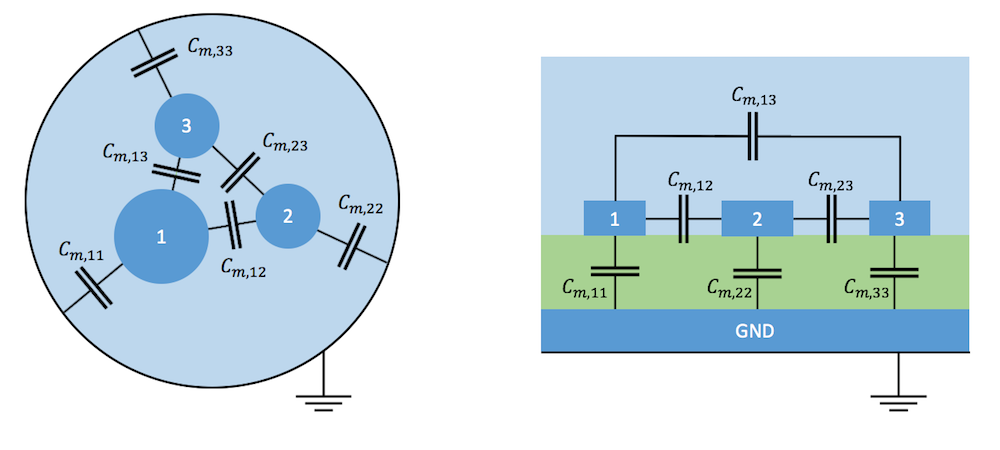Typical examples of mutual capacitance in a shielded three-conductor cable (left) and between microstrip transmission lines over a ground plate (right). The transition from a continuous field model to a lumped model with discrete capacitors means to shrink the conductors to points while moving the charges at their surface to the plates of capacitors shown between them.

For convenience, it is possible to arrange mutual capacitances of a system of N conductors and one additional ground in matrix form:

\begin{bmatrix}
C_{m,11} & C_{m,12} & \dots & C_{m,1N} \\
C_{m,21} & C_{m,22} & \dots & C_{m,2N} \\
\vdots & \vdots & \ddots & \vdots \\
C_{m,N1} & C_{m,N2} & \dots & C_{m,NN}
\end{bmatrix}.

The coefficients of this matrix, also called partial capacitances or lumped capacitances, are used in a circuit simulator when you reduce a physical system to a network of discrete elements.

In field theory, another matrix form is more common: the Maxwell capacitance matrix. Because the name is so similar and the coefficients are not identical, it is important to understand the relation between mutual and Maxwell capacitance matrices. The Maxwell capacitance matrix describes a relation between the charge of the ith conductor to the voltages of all conductors in the system.

\begin{pmatrix}
Q_1 \\
Q_2 \\
\vdots \\
Q_N
\end{pmatrix} =
\begin{bmatrix}
C_{11} & C_{12} & \dots & C_{1N} \\
C_{21} & C_{22} & \dots & C_{2N} \\
\vdots & \vdots & \ddots & \vdots \\
C_{N1} & C_{N2} & \dots & C_{NN}
\end{bmatrix}
\begin{pmatrix}
V_1 \\
V_2 \\
\vdots \\
V_N
\end{pmatrix}

The Maxwell capacitance matrix coefficient C_{11} could be determined by measuring the charge on conductor 1, when only the potential V_1=1 and all other electrodes are grounded. Therefore, the matrix is also often called the ground capacitance matrix. Its inverse, C^{-1}, is referred to as the elastance matrix.

We can also calculate the total charge on conductor 1 by summing up the contributions from the self- and mutual capacitances as follows.

\begin{matrix}
Q_1 &= &C_{m,11}V_1+C_{m,12}(V_1-V_2)+C_{m,13}(V_1-V_3)\\
&= & (C_{m,11}+C_{m,12}+C_{m,13})V_1-C_{m,12}V_2-C_{m,13}V_3)
\end{matrix}

For a system with N conductors, the relation between the mutual and Maxwell capacitance matrices is then

\begin{pmatrix}
Q_1 \\
Q_2 \\
\vdots \\
Q_N
\end{pmatrix} =
\begin{bmatrix}
\sum_{}{^N_{i=1}} C_{m,1i} & -C_{m,12} & \dots & -C_{m,1N} \\
-C_{m,21} & \sum_{}{^N_{i=1}} C_{m,2i} & \dots & -C_{m,2N} \\
\vdots & \vdots & \ddots & \vdots \\
-C_{m,N1} & -C_{m,N2} & \dots & \sum_{}{^N_{i=1}} C_{m,Ni}
\end{bmatrix}
\begin{pmatrix}
V_1 \\
V_2 \\
\vdots \\
V_N
\end{pmatrix}

You can easily tell a Maxwell capacitance matrix by its negative nondiagonal elements.

### Working Example: Mutual Capacitance of Two Spheres

Now that we have a clear definition of the terminology, let’s explore how easy it is to calculate capacitance matrices for arbitrary systems of conductors in COMSOL Multiphysics. To feel solid ground under our feet, we’ll start with a system with a known analytical solution. (Did I mention that I love analytical solutions? Actually, whenever I start a new simulation project, I try to find a simple system with an analytic solution to reproduce.)

In our case, we can use a system consisting of two conducting spheres of radii a and b, separated by a distance c and a ground reference at infinity.Closed-form expressions for such a system have been known since Maxwell’s times. I am referring to two readily available publications of de Queiroz (2003) and Lekner (2011). The expressions for the three Maxwell capacitance matrices are

C_{11}=Fab\sinh u \sum_{n=0}^\infty [a\sinh nu+ b\sinh (n+1)u]^{-1}
C_{22}=Fab\sinh u \sum_{n=0}^\infty [b\sinh nu+ a\sinh (n+1)u]^{-1}
C_{12}=-\frac{Fab}{c} \sinh u \sum_{n=1}^\infty [\sinh nu]^{-1}

where

You can easily declare these expressions as variables in COMSOL Multiphysics by using the sum operator:With a parametric sweep over N, we find that the series converges rapidly when the spheres are not too close to each other. We can safely set N to 10 for a given set of parameters: a = 0.1, b = 0.3, and c = 0.5.

In order to calculate the capacitance matrix in the Electrostatics interface, we set a terminal condition to one sphere with a potential of 1 V.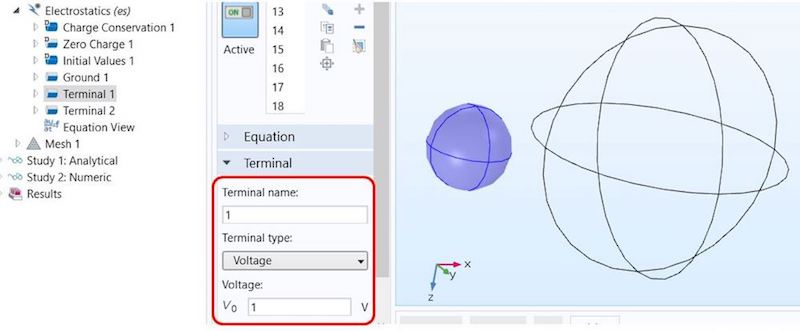Next, we duplicate the feature, apply it to the second sphere, and set the terminal name to 2. In order to calculate capacitance matrices, we need to apply different voltage or charge patterns to the terminals. For didactic reasons, we’ll discuss the traditional manual terminal sweep before introducing new, significantly faster technology that was released in version 5.3 of the COMSOL® software. While the new technology is faster in a large class of very common problems, the manual method is more general.

A manual terminal sweep is activated directly in the Electrostatics interface.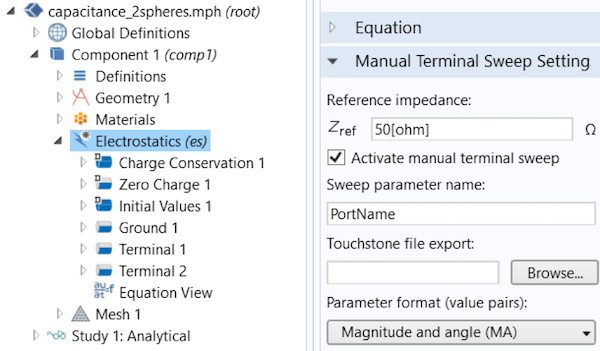After declaring the Sweep parameter name in the Global Parameters section (PortName by default), you can then run a parametric sweep over  PortName.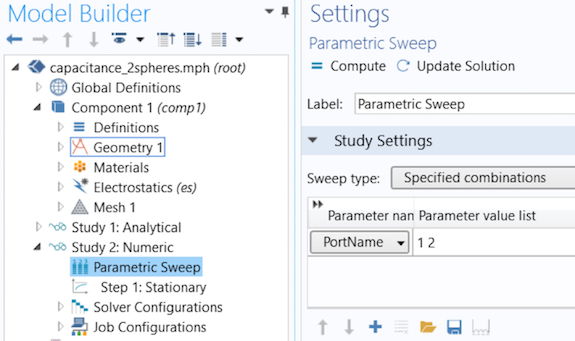In the model, the COMSOL Multiphysics software sets one terminal to 1 V and all others to ground during the sweep, resulting in the following two solutions: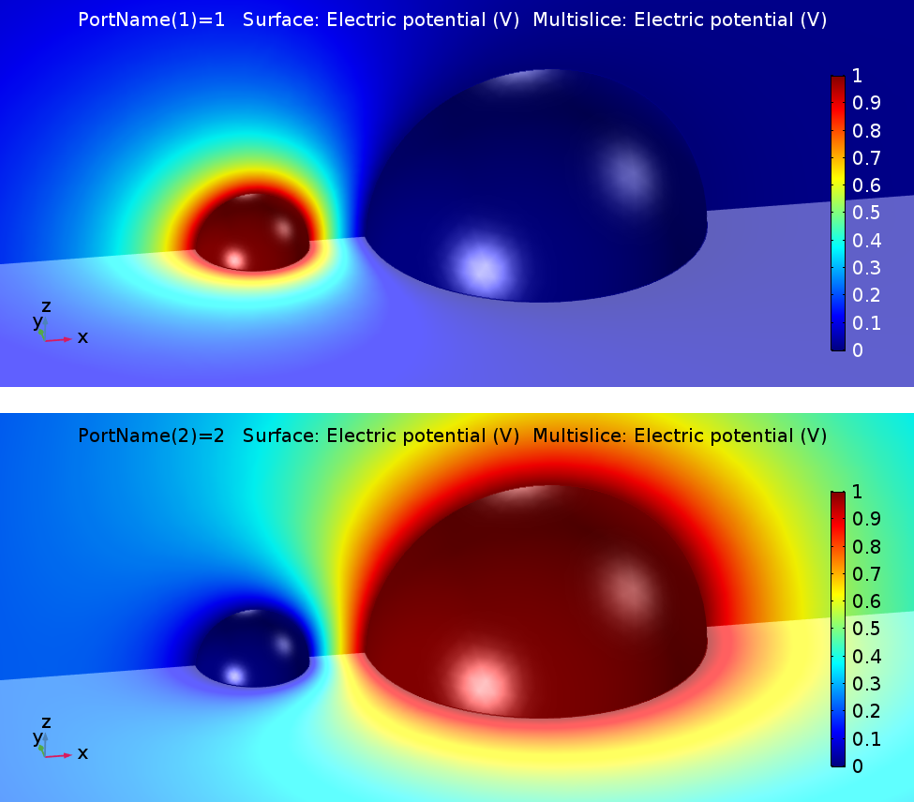You can use Results > Global Matrix Evaluation to extract the capacitance matrices in different notations, including the Maxwell capacitance matrix and the mutual capacitance matrix.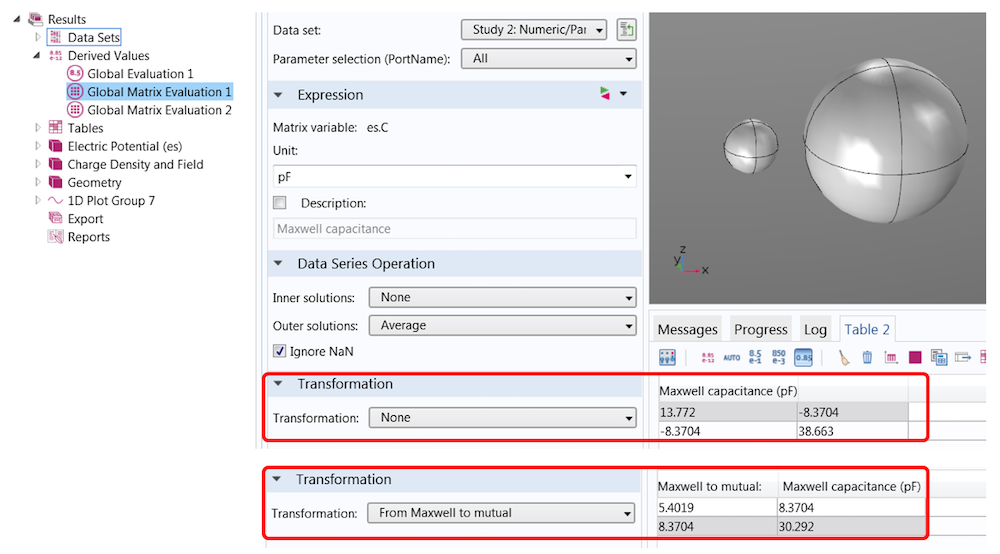In this simple example, their relation is

\begin{bmatrix}
C_{m,11} & C_{m,12} \\
C_{m,21} & C_{m,22}
\end{bmatrix}
=
\begin{bmatrix}
c_{11}+c_{12} & -c_{12} \\
-c_{21} & c_{22}+c{21}
\end{bmatrix}

If you are setting charge terminals instead of voltage terminals, the primary solution is the inverse capacitance matrix. A set of transformations are available that can help you convert the charge into the above-mentioned matrices.

### Gain Speed with Stationary Source Sweeps and the Boundary Element Method

In COMSOL Multiphysics version 5.3, we have introduced many powerful new modeling methods. One feature particularly relevant to capacitance matrix calculations is the new Stationary Source Sweep study step.

In contrast to manual terminal sweeps, which use a parametric sweep for PortName, this new technology accounts for the fact that applying different charges or voltage patterns to the electrostatic system doesn’t change the system matrix of the underlying FEM equations, only their loads. This means that the matrix needs to be inverted only once and can be reused for all other load cases. This approach can dramatically reduce computational time, especially when the number of terminals is large or other parametric sweeps (e.g., for the geometry) are needed.

Even for a moderate number of terminals, like in the Touchscreen Simulator simulation app, the speed gain is surprising: I could reach a factor of 7.8 on my machine for this model with the same number of DOF!

The new Capacitive Position Sensor tutorial model uses the Stationary Source Sweep study step.

A stationary source sweep is also easier to set up. There is no need to activate a manual terminal sweep and define a PortName variable and a parametric sweep. All you need to do is select a study step. By default, the study will run through all terminals. Alternatively, you can define specified sources that you want to cover.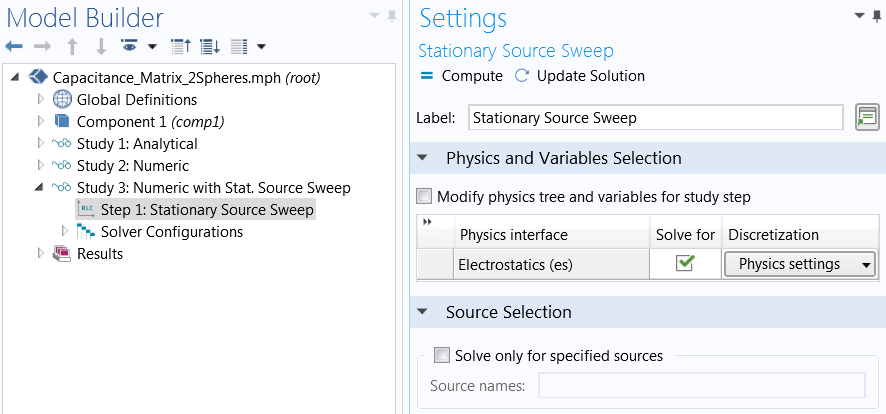If the stationary source sweep is so powerful, then why do we keep the traditional approach at all?

There are cases where we appreciate a recalculation of the system matrix; for instance, in nonlinear or multiphysics problems or if meshes should be adapted for each terminal configuration. In such cases, a manual terminal sweep is a better method.

Another powerful feature that comes with version 5.3 of the COMSOL® software is the boundary element method (BEM) in electrostatics. Compared to FEM, where a mesh in all domains (including the surrounding air domain) is needed, BEM avoids meshing in the infinite void, which reduces the number of DOFs. You can learn more about combining BEM in electrostatics and capacitance matrix calculation in the Modeling a Capacitive Position Sensor Using BEM tutorial.

### Concluding Thoughts on Capacitance Matrix Calculation

In this blog post, we have looked into the calculation of capacitance matrices, discussed the different terminologies, and provided a numerical solution for a well-known problem that has a closed-form solution. While the simple model presented here can provide a reference for your own models, the new features released in version 5.3 can help you to create large models with many terminals and additional parametric sweeps more efficiently.

• Browse these blog posts on capacitance and BEM:

### References

1. de Queiroz, A.C.M., 2003, “Capacitance Calculations“.
2. Lekner, J., 2011, “Capacitance coefficients of two spheres”, Journal of Electrostatics 69(1):11-14.

#### Categories##### LI WENZHENG
March 5, 2018

Hi Friedel,
Is there any way I can calculate the Capacitance Matrix by Time-Dependent mode? Because my model consists other stuff(neural activities) that is time-dependent. I’ve tried but there is no expression for Capacitance Matrix when the study is time-dependent.
Regards,
Wenzheng##### Sven Friedel
April 3, 2018

Dear Wenzheng,
Capacitance calculation is available only for stationary and frequency-dependent studies.
Imagine a RC situation. R and C can be uniquely determined from the real and imaginary parts of the impedance or admittance matrix. In a time-dependent situation this information is hidden in the U(t) and I(t) curves. See: https://www.comsol.ch/model/transient-modeling-of-a-capacitor-in-a-circuit-12695
In a situation where your system slowly changes in time you can replace the dependence on time t by a dependence on a parameter p and then possibly extract C(p).
Sven##### David Reens
May 1, 2018

Dear Sven,

What a great model and very useful for my research, thanks. There’s something strange to me about the conversion you show from a lumped/spice/mutual capacitance matrix to the maxwellian one. I think the former should have one fewer dimension than the latter owing to how the ground is treated. I believe a Maxwellian matrix should have rows and columns that sum to zero, no? Or is the convention here to leave off the last row and column of the Maxwell matrix, so that it gives vec{Q} = matrix(C)*vec{V} where the voltage and charge vectors exclude the ground?

Thanks,
Dave##### Sven Friedel
May 2, 2018

Dear Dave,
in fact we follow the convention vec{Q} = matrix(C)*vec{V}, where ground is excluded. If you look at fig. 1 above, you see a system of 3 conductors (+ one ground at infinity, or surrounding shield). This makes in total N=4 poles. The capacitance matrix has N*(N-1)/2 coefficients, in this case 6, which can be expressed by a symmetric (N-1)x(N-1) matrix.
Best regards
Sven##### 岩 李
June 25, 2018

I have a four core cable and I want to get the capacitance matrix over frequency.
I set the common screen to be ground and set the four cores to be different terminal, list them 1 to 4. and set them to be voltage terminal type with voltage of 1V.
I want to use parameter sweep of PortName. However, I am confused about how to define PortName in global parameter definition. I can give a name of PortName, but then what is the expression? I now set it to be PortName again and I get yellow warning. Simulation seems to run but I get nothing in result.
Another question is how can I get the result in a frequency range?
Thanks##### Gleb Kuman
May 17, 2019

Dear, Sven
Thanks you a lot for such nice blog post!

– According to equation Q1 = Cm11V1 + Cm12(V1 – V2) + etc, if we delete all conductors from the model beside 1st, we obtain simple equation for capacity of single conductor Q1 = Cm11*V1.
– Consequently capacity of first sphere Cm11, that in your example equals 5.4pF (form mutual matrix) should be equal to single sphere capacity, that according to C = 4*pi*Eps0*r equals 11.3pF.
– Can you tell please what the reason of this difference? Why Cm11 dont equals capacity of single sphere?
Thanks##### Sven Friedel
May 17, 2019

The diagonal elements of the mutual capacitance matrix are indeed not identical with the self-capacitance of the respective body. Let us refer to the original definition “capacitance describes the amount of charge that a body needs in order to raise its electric potential by 1 volt against a grounded reference potential.”
If, besides the ground at infinity, another body is present (also grounded), then the amount of charge that needs to be stored in order to raise its potential to 1 V is less. The diagonal elements of the capacitance matrix are defined “in presence” of the other body, this is also obvious from the analytical description C11 is a function of a and b. For b–>0 you get the sel-capacitance of the sphere with radius a.

lim_b->0( F*a*b*sinh(u)*sum(1/(a*sinh(n*u)+b*sinh((n+1)*u)),n,0,N) ) = 4*pi*epsilon0_const*a

Check it out!
Sven##### Gleb Kuman
May 17, 2019

Dear, Sven
Thank you very much for such full and quick response.
Now it all clear!

Regards, Gleb##### Oscar Diaz
February 4, 2021

Hi Friedel,
Really interesting blog on mutual capacitance calculation. I run both methods explained: 1) manual terminal sweep and 2) stationary source sweep. With the 1st one, the resulting solved model is heavier and the mutual capacitance matrix can be obtained without a problem. For the 2nd one I have not been able to extract the matrix, since every time I try to do the global matrix evaluation, the output returns only ‘NaN’ cells.
Thanks!##### Sven Friedel
February 4, 2021 COMSOL Employee

Dear Oscar – I would reciommend you creating a regular support case. There we can help you best.##### Prasanna Kumar Routray
July 29, 2021

Hi Sven,
Do you have the .mph file for this tutorial?

EXPLORE COMSOL BLOG